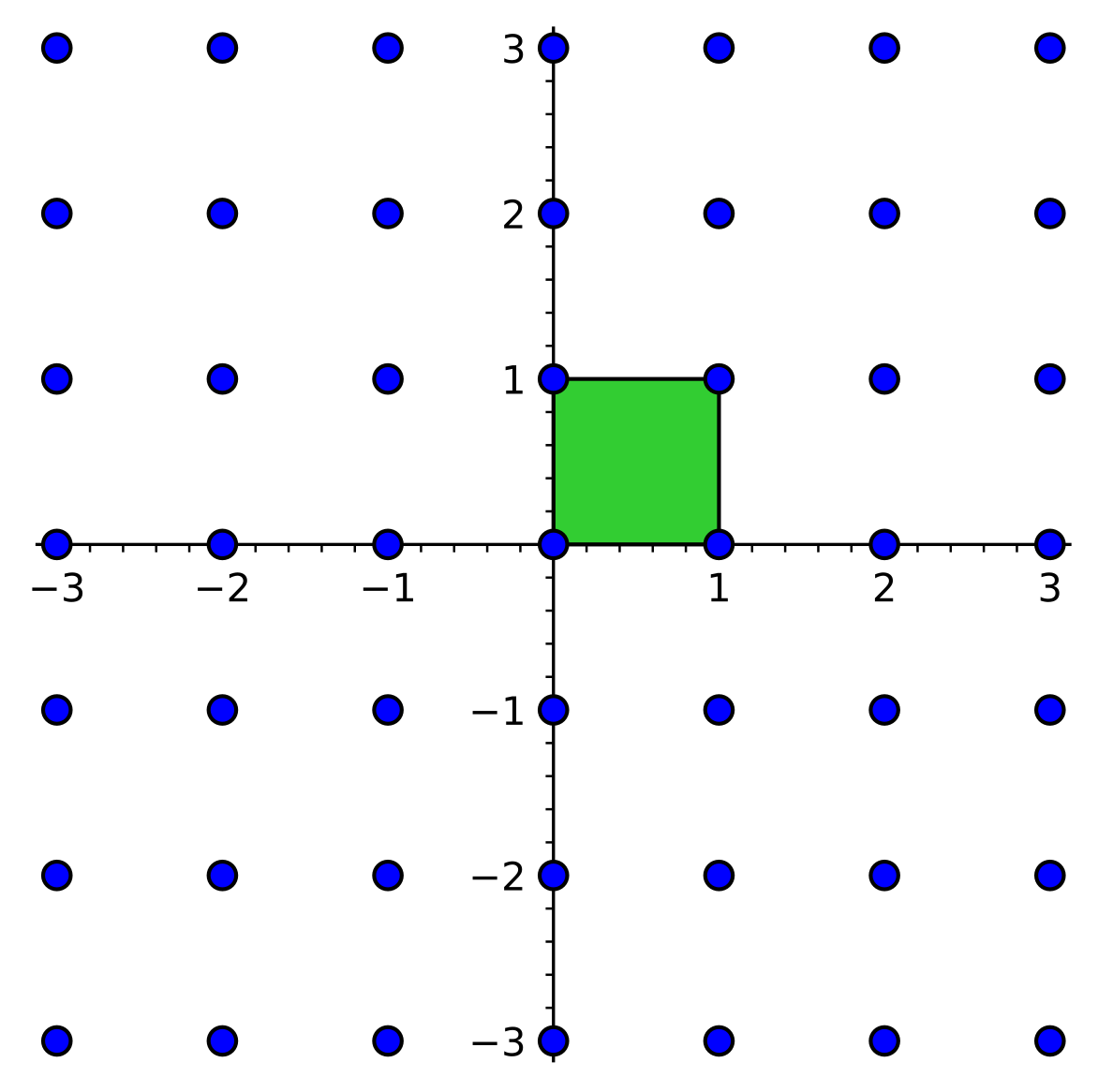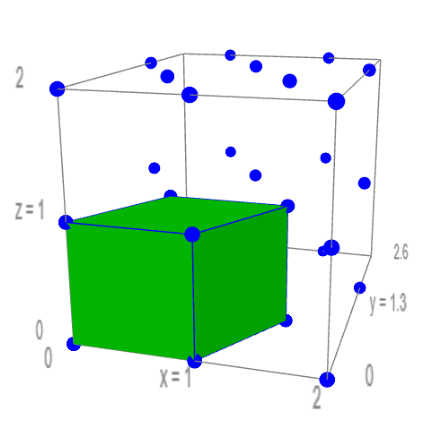# Eight-dimensional spheres and the exceptional $E_8$

What is the $E_8$ lattice that appears in Viazovska's proof? What makes it special? How do you use it to pack spheres?

# Eight-dimensional spheres and the exceptional $E_8$

Ursula Whitcher
Mathematical Reviews (AMS)

In Helsinki this summer, Ukrainian mathematician Maryna Viazovska was awarded a Fields Medal "for the proof that the $E_8$ lattice provides the densest packing of identical spheres in 8 dimensions, and further contributions to related extremal problems and interpolation problems in Fourier analysis."Maryna Viazovska at the Oberwolfach research institute in 2013. Photo by Petra Lein (CC BY-SA 2.0 DE).

Finding the most efficient way to pack identical spheres is an extremely challenging problem! Even in three dimensions, this was an open question for hundreds of years. Johannes Kepler asserted that the familiar stacked pyramid was the best possible option in the seventeenth century, but the first person to provide a complete proof of this fact was Thomas Hales, in 1998. (Bill Casselman illustrated some of Hales' arguments using two-dimensional examples in the December 2000 Feature Column, Packing pennies in the plane.)Freshly baked rolls approximate the best three-dimensional sphere packing. Photo by AJ Lanphere.

In 2016, Viazovska found an elegant way to show that a particular method of packing spheres in 8-dimensional space was the best. She then teamed up with four other mathematicians inspired by her arguments, Henry Cohn, Abhinav Kumar, Stephen D. Miller, and Danylo Radchenko, to prove a similar result in 24 dimensions. Six years later, 8 and 24 are still the only dimensions higher than 3 where the best way to pack identical spheres is known! The best way to pack spheres in these dimensions turns out to be extremely symmetrical. This might not be true in every dimension—maybe sometimes it's better to squeeze extra higher-dimensional spheres into unexpected corners!

What is the $E_8$ lattice that appears in Viazovska's proof? What makes it special? How do you use it to pack spheres? Let's explore these questions and check out some beautiful visualizations of $E_8$.

### Lattices

Before we define $E_8$, we should explore the more general concept of a lattice. It's important to be specific here, because the word "lattice" is used for multiple distinct mathematical concepts! The set of points in the plane with integer coordinates is an easy-to-visualize example of the lattices we'll be talking about today.There are lots of different ways to describe the points in the plane with integer coordinates. One place to start is to focus on the points $(0,1)$ and $(1,0)$. If we add these points together, we get a new point, $(1,1)$, that also has integer coordinates. If we keep on adding or subtracting $(0,1)$ and $(1,0)$, we will eventually reach every point in the plane with integer coordinates!

We can connect the origin $(0,0)$, the starting points $(0,1)$ and $(1,0)$, and the first sum $(1,1)$ to make a square. A square is a special kind of parallelogram, and this square is called the fundamental parallelogram for the lattice of points with integer coordinates.Now, what if we reversed the procedure? Instead of starting with an infinite set of scattered points and making a parallelogram, we could start with a parallelogram and create an infinite set. Our procedure is to place one vertex of the parallelogram at the origin, then repeatedly add or subtract the points corresponding to the two vertices of the parallelogram adjacent to the origin. In particular, the sum of these two vertices is the remaining vertex of the parallelogram.In three dimensions, we could generalize this procedure using the corners of a box. Or more broadly, since we don't need the edges to meet at right angles, we could use the three-dimensional generalization of a parallelogram: a parallelepiped. (Here's an etymological puzzle I learned from John Conway: why don't we pronounce "parallelepiped" as "parallel-epi-ped," emphasizing the Greek root for "on"?)A perspective view of a three-dimensional lattice

To build a lattice in $n$ dimensions, we just need the $n$-dimensional generalization of a parallelogram and parallelepiped. Such a shape is called a parallelotope. Alternatively, we could simplify a bit by focusing on the $n$ vertices of our fundamental parallelotope that are connected by a line segment to the origin. (Linear algebra enthusiasts will recognize that we are specifying a basis of $\mathbb{R}^n$.)

### The points of $E_8$

We are ready to describe the $E_8$ lattice! Concretely, it is the eight-dimensional lattice determined by the eight following fundamental parallelotope vertices:

• $(1,-1,0,0, 0,0,0,0)$
• $(0,1,-1,0, 0,0,0,0)$
• $(0,0,1,-1, 0,0,0,0)$
• $(0,0,0,1, -1,0,0,0)$
• $(0,0,0,0, 1,-1,0,0)$
• $(0,0,0,0, 0,1,-1,0)$
• $(0,0,0,0, 0,1,1,0)$
• $(-\frac{1}{2},-\frac{1}{2},-\frac{1}{2},-\frac{1}{2}, -\frac{1}{2},-\frac{1}{2},-\frac{1}{2},-\frac{1}{2})$.

Repeatedly adding and subtracting these points creates the entire infinite $E_8$ lattice.

You might notice that the points of $E_8$ have either integer coordinates or half-integer coordinates, but never a combination of integers and half-integers. Another way to describe $E_8$ is that it consists of the points in eight dimensions with only integer or only half-integer coordinates where the sum of the coordinates is an even number.

Maryna Viazovska showed that the most efficient sphere packing in eight dimensions places a sphere center at each of the points in the $E_8$ lattice. What is the radius of these spheres? You might notice that each of the eight fundamental parallelotope vertices we used to build $E_8$ is a distance of $\sqrt{2}$ from the origin. This turns out to be the smallest possible distance between any pair of points in the $E_8$ lattice, so if we give each sphere a radius of $\sqrt{2}/2$, the spheres will touch without overlapping. (Of course, if we want to pack identical eight-dimensional spheres that have some other radius, we can scale the entire picture up or down.)

The eight special points we chose are not the only points in $E_8$ with minimum distance from the origin! We can quickly construct eight more by subtracting our chosen points from the origin. But that is only the beginning. There are 240 points in $E_8$ at a distance of $\sqrt{2}$ from the origin. These special points are called roots. We can immediately see that in the $E_8$ lattice packing, a sphere centered at the origin will touch 240 other spheres. Because we can move any point of $E_8$ to the origin by subtracting its coordinates from every other lattice point, we conclude that every sphere in the $E_8$ lattice packing touches 240 other spheres.

We can use the 240 roots of $E_8$ to construct a polytope—a higher-dimensional polyhedron—that has 240 vertices. Formally, this polytope is known as the $4_{21}$ polytope, based on a classification by the lawyer and amateur mathematician Thorold Gosset. Because it lives in eight dimensions, we can't visualize the $4_{21}$ polytope directly. However, we can create images describing which vertices are connected by edges to which other vertices, in a process analogous to the diagrams of a cube you might draw on a sheet of paper. This helps us visualize the structure of the 240 spheres surrounding the sphere at the origin in the $E_8$ lattice packing.

Here is a projection of the $E_8$ root polytope into three dimensions:Visualization by J.G. Moxness (CC BY-SA 3.0).

The mathematician José Luis Rodríguez Blancas led a project to visualize the $4_{21}$ polytope using colored thread. In this visualization, the 240 roots are divided into eight different "crowns," each containing 30 vertices.Thread art by José Luis Rodríguez Blancas (CC BY-SA 3.0).

### Inner products and the magic of 8

Another way to understand the eight fundamental parallelotope vertices connected to the origin is to look at the angle described by the origin and each pair of vertices. Let's think of the vertices as vectors with their heads at the vertex and the tail at the origin. If we measure in radians, the angle $\theta$ between any pair of vectors $\mathbf{v}$ and $\mathbf{w}$ satisfies the equation

$$\cos \theta = \frac{\mathbf{v} \cdot \mathbf{w}}{||\mathbf{v}|| ||\mathbf{w}||}.$$

In our eight-dimensional case, $(v_1, \dots, v_8) \cdot (w_1, \dots, w_8) = v_1 w_1 + \dots v_8 w_8$ and the length $||(v_1,\dots,v_8)||$ is given by $\sqrt{v_1^2+\dots+v_8^2}$. Because each of our eight special vertices is a distance of $\sqrt{2}$ from the origin, we know $||\mathbf{v}|| ||\mathbf{w}|| = 2$ for any pair of special vertices, so

$$\cos \theta = \frac{1}{2} \mathbf{v} \cdot \mathbf{w}.$$

Thus, we can determine the angles between pairs of our special vertices by calculating their dot products. Here's a matrix with every possible pair of dot products:

$$\begin{pmatrix} 2 & -1 & 0 & 0 & 0 & 0 & 0 & 0 \\ -1 & 2 & -1 & 0 & 0 & 0 & 0 & 0 \\ 0 & -1 & 2 & -1 & 0 & 0 & 0 & 0 \\ 0 & 0 & -1 & 2 & -1 & 0 & 0 & 0 \\ 0 & 0 & 0 & -1 & 2 & -1 & -1 & 0 \\ 0 & 0 & 0 & 0 & -1 & 2 & 0 & 0 \\ 0 & 0 & 0 & 0 & -1 & 0 & 2 & -1 \\ 0 & 0 & 0 & 0 & 0 & 0 & -1 & 2 \end{pmatrix}$$

We have 2s on the diagonal, as expected. Distinct vertices have dot product either 0 or -1, so the corresponding angles are either right angles or $2 \pi/3$ ($120^\circ$)—a strikingly symmetrical arrangement!

A subtler fact about this matrix of dot products is that it has determinant 1. When a lattice's dot product matrix has this property, we say that the lattice is unimodular. In any dimension, the lattice of all points with integer coordinates is unimodular, since the corresponding dot product matrix is the identity matrix. But every point in the $E_8$ lattice has an even dot product with itself, and even unimodular lattices are much rarer. In fact, $E_8$ is the smallest even unimodular lattice—as long as we assume that our lattices can be embedded in $\mathbb{R}^n$! (If you're willing to admit imaginary lengths, your options for building unimodular objects expand.)

We can make a sixteen-dimensional even unimodular lattice by taking pairs of lattice points in $E_8$. There's a second even unimodular lattice in dimension sixteen, as well. But there are no other geometrically feasible options between 8 and 16. The next even unimodular lattices appear in 24 dimensions. That's why Viazovska and her collaborators guessed there should be a way to extend her techniques from 8 to 24-dimensional sphere packings—skipping everything in between!